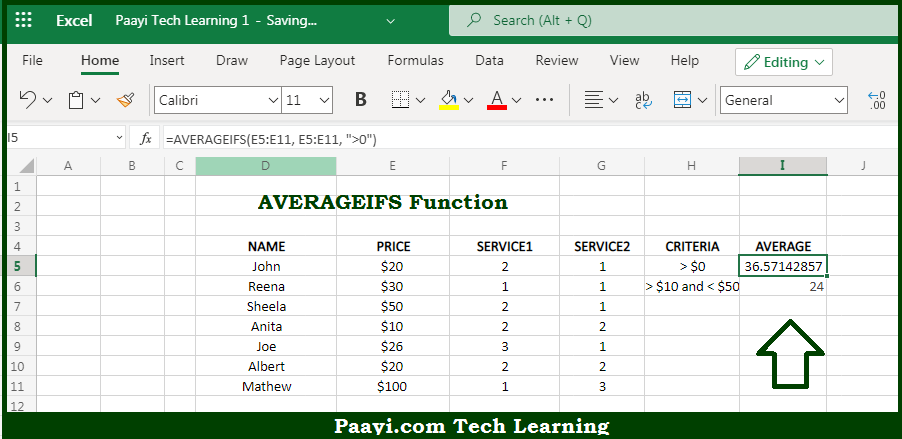# Learn How to Use Microsoft Excel AVERAGEIFS Function

Written by | 0 Comments | 642 Views

In this article, you will learn how to use the Microsoft Excel AVERAGEIFS function and its prime function in Microsoft Excel. You will also get to know the Microsoft Excel AVERAGEIFS function return value and syntax with the help of some examples.

Microsoft Excel AVERAGEIFS Function

The main purpose of the Microsoft Excel AVERAGEIFS function is to get the average of cells that match multiple criteria. That implies, with the help of the AVERAGEIFS function you can able to calculate the average of numbers in a range that meets one or more supplied criteria. The AVERAGEIFS criteria can include logical operators like (>,<,<>,=) and wildcards (*,?) for partial matching. So, with the help of the AVERAGEIFS function, you can able to get the average of cells provided that meet multiple criteria.

Return Value of AVERAGEIFS Function

The return value will be the average of cells that meet all the criteria.

Syntax of AVERAGEIFS Function

=AVERAGEIFS(avg-rng, range1, criteria1, [range2], [criteria2], ...)

Where the arguments:

• avg-rng: This is the range to average.
• range1: This is the first range to evaluate.
• criteria1: This is the criteria to use on range1.
• range2: This is the second range to evaluate (optional).
• criteria2: This is the criteria to use on range2 (optional).

## How to Use Microsoft Excel AVERAGEIFS Function?So we know that Microsoft Excel AVERAGEIFS function you can able to get the average of cells that match multiple criteria. That implies, with the help of the AVERAGEIFS function you can able to calculate the average of numbers in a range that meets one or more supplied criteria. The AVERAGEIFS criteria can include logical operators like (>,<,<>,=) and wildcards (*,?) for partial matching. So, with the help of the AVERAGEIFS function, you can able to get the average of cells provided that meet multiple criteria.